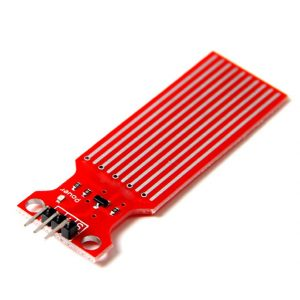# WATER LEVEL MEASUREMENT USING ARDUINO UNO R3 AND WATER SENSORS

Hello , Welcome back , In this tutorial we are going to show you how to make a water level indicator using arduino uno and some water sensors . There are so many tutorial on water level sensors on internet , but this method is some thing different than other . First of all let us discuss about component we need to make water level indicator and controller.

## Component required to make water level indicator .

water sensors that can give output 3-5 volt when water is present -8
Light Emitting Diodes (LEDs) -4
arduino uno or similar
buzzer
jumper wires
Liquid Crystal Display (LCD)
motor
relay with power source
tip-122 NPN transistor
power source

First of all let us discuss about water sensor . Since there are so many water sensors available in the market we can use any one of them that gives output 1 when that sensor is drown into water ( Since 100% pure water is good insulator , but it is hard to find water which is 100% pure so , basically water conducts electricity , , if water conducts electricity you can give 5 volt to the bottom of the water so that if water reach upper levels respective conductive pins goes to high and hence water detected ).
or alternatively you can use water sensors as shown in figure below and it will produce 4.3 volt if complete drowned to water which means 1 in digital electronics .This is analog water sensor but we can use this sensor as digital if its strips are complete drowned it will give logic 1 ,

If you are not familier about other components please check arduino tutorials for beginners and you will learn something new before exploring other tutorial .

Okay now connect everythings as shown in circuit diagram and upload the code as shown in below and after that we will describe the code brifly .You can download the complete code , simulation file , from this link water level indication electronify.

## WATER LEVEL MEASUREMENT USING ARDUINO UNO R3 AND WATER SENSORS (Code)

``` #include LiquidCrystal lcd(13, 12, 11, 10, 9, 8); byte sensorPin[] = {0,1,2,3,4,5,6,7}; byte ledPin[] = {17,16,15,14}; // number of leds = numbers of sensors const byte sensors = 8; const byte leds=4; int motor = A4; int buzzer = A5; void setup() { for(int i = 0; i< sensors; i++) //initialize all sensor pins to input pin { pinMode(sensorPin[i], INPUT); } for(int i = 0; i< leds; i++) //initialize all led pins to output pins { pinMode(ledPin[i], OUTPUT); } pinMode(motor, OUTPUT); //initialize motor pin to output pin lcd.begin(20, 4); //initializing 20x4 LCD lcd.clear(); //clear LCD first lcd.print("ELECTRONIFY.ORG"); // display string electronify.org } void loop() { int level = 0; for(int i = 0; i < sensors; i++) { if(digitalRead(sensorPin[i]) == HIGH) { digitalWrite(ledPin[i/2], HIGH); level = i+1; } else { digitalWrite(ledPin[i/2], LOW); } } lcd.setCursor(0,1); lcd.print("WATER LEVEL MEASURE"); lcd.setCursor(0,2); switch(level) { case 1: lcd.print("LEVEL 1"); digitalWrite(motor, HIGH); break; case 2: lcd.print("LEVEL 2"); digitalWrite(motor, HIGH); break; case 3: lcd.print("LEVEL 3 "); digitalWrite(motor, HIGH); break; case 4: lcd.print("LEVEL 4 "); digitalWrite(motor, LOW); break; case 5: lcd.print("LEVEL 5 "); digitalWrite(motor, LOW); break; case 6: lcd.print("LEVEL 6 "); digitalWrite(motor, LOW); break; case 7: lcd.print("LEVEL 7 "); digitalWrite(motor, LOW); break; case 8: lcd.print("LEVEL 8 "); digitalWrite(motor, LOW); lcd.setCursor(0,3); lcd.print("WARNING : OVERFLOW"); for(int j = 0; j< 255; j++) { analogWrite(buzzer, j); delay(10); } break; default: lcd.print("NO WATER"); digitalWrite(motor, HIGH); break; } delay(50); } ```

## WATER LEVEL MEASUREMENT USING ARDUINO UNO R3 AND WATER SENSORS (Schematic Diagram)# Short Description

The code is very simple to understand . Since we are using 8 water sensors on PORTD so all of the pins on PORTD was initialized as output PORT , similarly LCD and other peripherals are initialized . After that input is taken from the water sensors , and if it produce output1 corresponding level is displayed on the LCD . Also motor is turned on for level 0-3 , and warning message is displayed on water level 8 .